# RF Tutorial Lesson 11: Designing a Microstrip MESFET Amplifier

 Tutorial Project: Designing a Microstrip MESFET Amplifier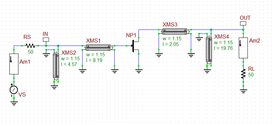Objective: In this project, you will build and test a distributed RF amplifier using your own S-parameter-based MESFET model. Concepts/Features: RF Amplifier S-Parameter-Based MESFET Model Maximum Gain Design Microstrip Line Segment AC Frequency Sweep Power Gain Minimum Version Required: All versions

## What You Will Learn

In this tutorial you will learn how to import an RF FET model from a text file and will build a distributed RF amplifier using a unilateral MESFET along with physical microstrip components for the input and output matching networks.

## Importing High Frequency MESFET Model

In Tutorial Lesson 10, you learned how to create a new RF BJT device. For this tutorial lesson, you need to create an RF MESFET device. The measured data for the MESFET device are given below:

f(GHz) s11 s21 s12 s22
3.0 0.80 ∠ -90 ° 2.80 ∠ 100 ° 0 0.66 ∠ -50 °
4.0 0.75 ∠ -120 ° 2.50 ∠ 80 ° 0 0.60 ∠ -70 °
5.0 0.71 ∠ -140 ° 2.30 ∠ 60 ° 0 0.58 ∠ -85 °

Open RF.Spice's Device Manager and select "Create New RF Device from S-Parameter Test File..." from its File Menu. Follow the program's prompts step by step and create your new MESFET devices according to the table below:

File Name Model Name Symbol Name Symbol
MyMESFET.txt MyMESFET mesfet_n## Building & Testing a Distributed MESFET Amplifier with Microstrip Components

The following is a list of parts needed for this part of the tutorial lesson:

Part Name Part Type Part Value
VS AC Voltage Source 1V
NP1 N-Type RF MESFET Imported Model: MyMESFET
XMS1 Microstrip Line w = 1.15, h = 0.5, er = 3.4. len = 8.19
XMS2 Microstrip Line w = 1.15, h = 0.5, er = 3.4. len = 4.57
XMS3 Microstrip Line w = 1.15, h = 0.5, er = 3.4. len = 2.05
XMS4 Microstrip Line w = 1.15, h = 0.5, er = 3.4. len = 19.76
RS, RL Resistor 50The property dialog of the MESFET device.

The goal of this part is to design a distributed MESFET amplifier with a gain of 11dB at f = 4GHz. From the S-parameter data of the MESFET, we know that it is a unilateral transistor, i.e. s12 = 0. Moreover, |s11| < 1 and |s22| < 1. Therefore, the MESFET is unconditionally stable. This reduces the input and output reflection coefficients to:

$\Gamma_{in} = s_{11}$

$\Gamma_{out} = s_{22}$

The conjugate matching conditions at the input and output of the unilateral transistor reduce to:

$\Gamma_S = s_{11}^{\ast}$

$\Gamma_L = s_{22}^{\ast}$

Furthermore, you have:

$G_0 = |s_{21}|^2 = 6.25 = 8.0dB$

$G_{Tmax} = G_S . G_0 . G_L = \frac{1}{1- |s_{11}| ^2} . |S_{21}|^2 . \frac{1}{1- |s_{22}| ^2} = 13.5dB$

To achieve a gain of 11dB, you have 2.5dB more available gain. So you set GS = 2dB and GL = 1dB for a total gain of GT = 2dB + 8dB + 1dB = 11dB. The complex value of ΓS is found on the constant circle GS = 2dB, and the complex value of ΓL is found on the constant circle GL = 1dB, in both cases trying to minimize the distance from the center of the Smith Chart. This requirement yields:

ΓS = 0.33 ∠120°

ΓL = 0.22 ∠70°

For your MESFET amplifier, you will use the same input and output matching network types as in Tutorial Lesson 10, consisting of a 50Ω transmission line segment together with a shunt 50Ω Open Stub.

For this project, you will use a thin lossless dielectric substrate of thickness h = 0.5mm and relative permittivity &epsilonr = 3.4. A 50Ω microstrip line on this substrate has a width of 1.15mm. At the design frequency of f = 4GHz, the guide wavelength of this microstrip line is λg = 45.74mm. The lengths of the microstrip segments are found to be:

Microstrip Component Z0 Electrical Length Physical Width Physical Length
XMS1 50Ω 0.179λg 1.15mm 8.19mm
XMS2 50Ω 0.100λg 1.15mm 4.57mm
XMS3 50Ω 0.045λg 1.15mm 2.05mm
XMS4 50Ω 0.432λg 1.15mm 19.76mm

Place and connect all the parts as shown in the figure below. For the shunt stubs, connect the microstrip segments XMS2 and XMS4 in a parallel fashion between the source and load resistors and the ground, respectively. Leave the positive pin of XMS2 and XMS4 open.The MESFET Amplifier without the source and load sections for the purpose of network analysis.

Run a Network Analysis Test of this circuit according to the table below:

 Start Frequency 3G 5G 10Meg Linear S Smith or Cartesian (Amplitude Only) with Decibels checked

The figure below shows the results for S11, S21, S12 and S22 parameters. Note that since S12 = 0, its dB-scale plot falls at a very large negative number. Therefore, you need to adjust the scale of the vertical axis. Or you can deselect S12 from the graph's legend and zoom to fit. The insertion gain |s21| is almost 11dB as expected from the design. However, the value of the return loss |s11| is only -5dB and certainly not very good. This is due to the fact that you had to deliberately introduce a mismatch in the input and output matching networks to achieve the specified gain of 11dB.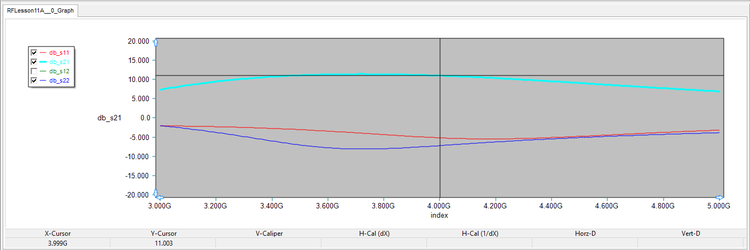The graph of magnitude of S11, S21 and S22 parameters of the MESFET amplifier circuit (S12 = 0).

## Running an AC Frequency Sweep to Compute Power Gain

Next, connect the AC voltage source and the source and load resistors and place two ammeters at the source and load ammeters in a similar manner as in the last part of Tutorial Lesson 10. The figure below shows the circuit with the source, load and ammeters: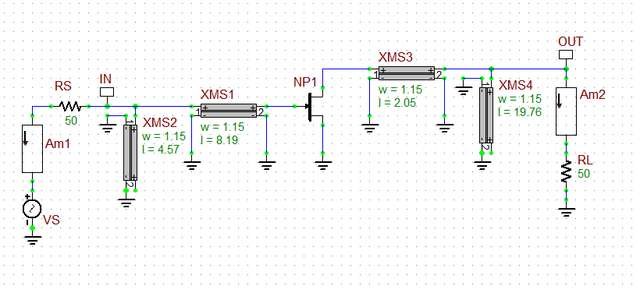The MESFET amplifier with microstrip matching networks at its input and output.

Run an AC Frequency Sweep Test of your amplifier according to the table below:

 Start Frequency 3G 5G 10Meg Linear i(iam1), i(iam2)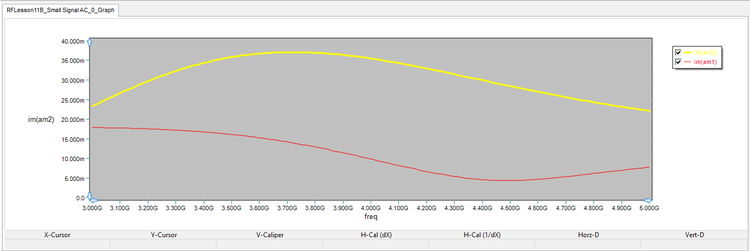The graph of variation of input source current and output load current as a function of frequency.

Also define a custom output plot called "Power_Gain" for your amplifier using the same definition: GP = 20*log10(abs(i(am2)/i(am1))).

 Start Frequency 3G 5G 10Meg Linear Custom: Power_Gain

The figure below shows the graph of power gain vs. frequency. Using the crosshairs you can read the value of the power gain at 4GHz to be 11.148dB, which agrees well with the value of insertion gain calculated in the previous part.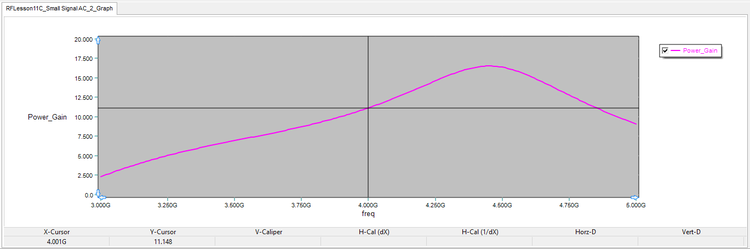The graph of the power gain of the MESFET amplifier vs. frequency.